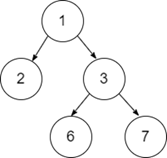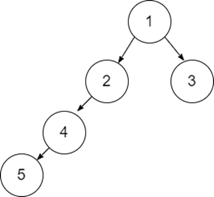# Balanced Binary Tree in Python

In a binary tree, each node contains two children, i.e left child and right child. Let us suppose we have a binary tree and we need to check if the tree is balanced or not. A Binary tree is said to be balanced if the difference of height of left subtree and right subtree is less than or equal to '1'.

Example

Input-1:Output:

True

Explanation:

The given binary tree is [1,2,3, NULL, NULL, 6, 7]. The height difference of its left subtree and right subtree is equal to '1', thus it is a height Balanced tree.

Input-2:Output:

False

Explanation:

The given binary tree is [1,2,3,4, NULL, NULL,NULL,5]. The height difference of its left subtree and right subtree is greater than '1', thus it is not a height balanced tree.

## Approach to Solve this Problem

The recursive approach to solve this problem is to find the height of the left subtree and the right subtree and then check if (height(leftsubstree) - height(rightsubtree) <= 1) and return True or False accordingly. Then, we will check recursively for each node of the binary tree.

• Take input of nodes of a Binary Tree.
• Define a function to find the height of the tree.
• A Boolean function to check recursively if the height difference of left subtree and right subtree is not more than '1', then return True.
• Return the Result.

## Example

Live Demo

class treenode:
def __init__(self, data):
self.data = data
self.left = self.right = None
# funtion to find the height of the left subtree and right subtree
class height:
def __init__(self):
self.height = 0
# function to check if the tree is balanced or not
def isBalanced(root):
lh = height()
rh = height()
if root is None:
return True
return (
(abs(lh.height - rh.height) <= 1)
and isBalanced(root.left)
and isBalanced(root.right)
)
root = treenode(1)
root.left = treenode(2)
root.right = treenode(3)
root.left.left = None
root.left.right = None
root.right.left = treenode(6)
root.right.right = treenode(7)
if isBalanced(root):
print("Balanced")
else:
print("Not Balanced")

Running the above code will generate the output as,

## Output

Balanced

The given binary tree [1, 2, 3, NULL, NULL, 6, 7]. The height difference of its left subtree and right subtree is equal to '1', thus it is a height Balanced tree.